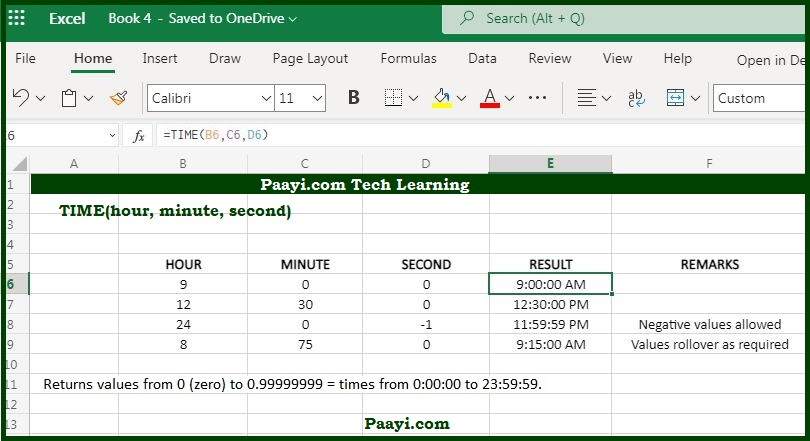# Learn How to Use Microsoft Excel TIME Function

Written by | 0 Comments | 668 Views

In this article, you will learn how to use the Microsoft Excel TIME function and its prime function in Microsoft Excel. You will also get to know the Microsoft Excel TIME function return value and syntax with the help of some examples.

## Microsoft Excel TIME Function

The TIME function's main purpose is to create a time with hours, minutes, and seconds. So, it means with the Microsoft Excel TIME function; you can create a time with hour, second and minute components. The built-in TIME function can be used when you want to assemble a proper time inside a formula.

### TIME Function Return Value

It will return a decimal number representing a particular time in Excel.

### TIME Function Syntax

= TIME(hour, minute, second)

Where:

• hour: The hour component for the time you want to create.
• minute: The minute component of time you want to create.
• second: The second component of time you want to create.

## How to Use Microsoft Excel TIME Function?As you know, the TIME function creates a date in a serial number format from the hour, minute, and second components supplied by you. Let us go through some of the examples of the TIME function as given below:

• =TIME(5, 0, 0) - This will return the 5 hours.
• =TIME(0, 5, 0) - This will return the 5 minutes.
• = TIME(0, 0, 5) - This will return the 5 seconds.
• = TIME(9, 30, 0) - This will return the 9.5 hours.